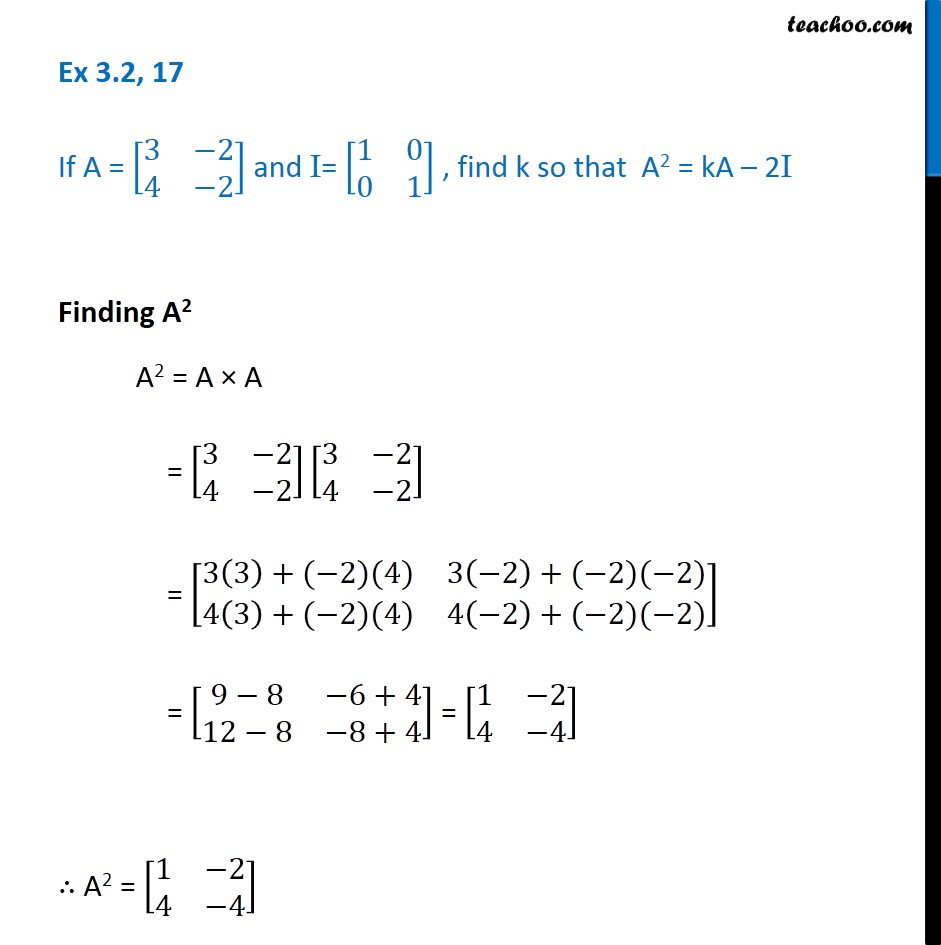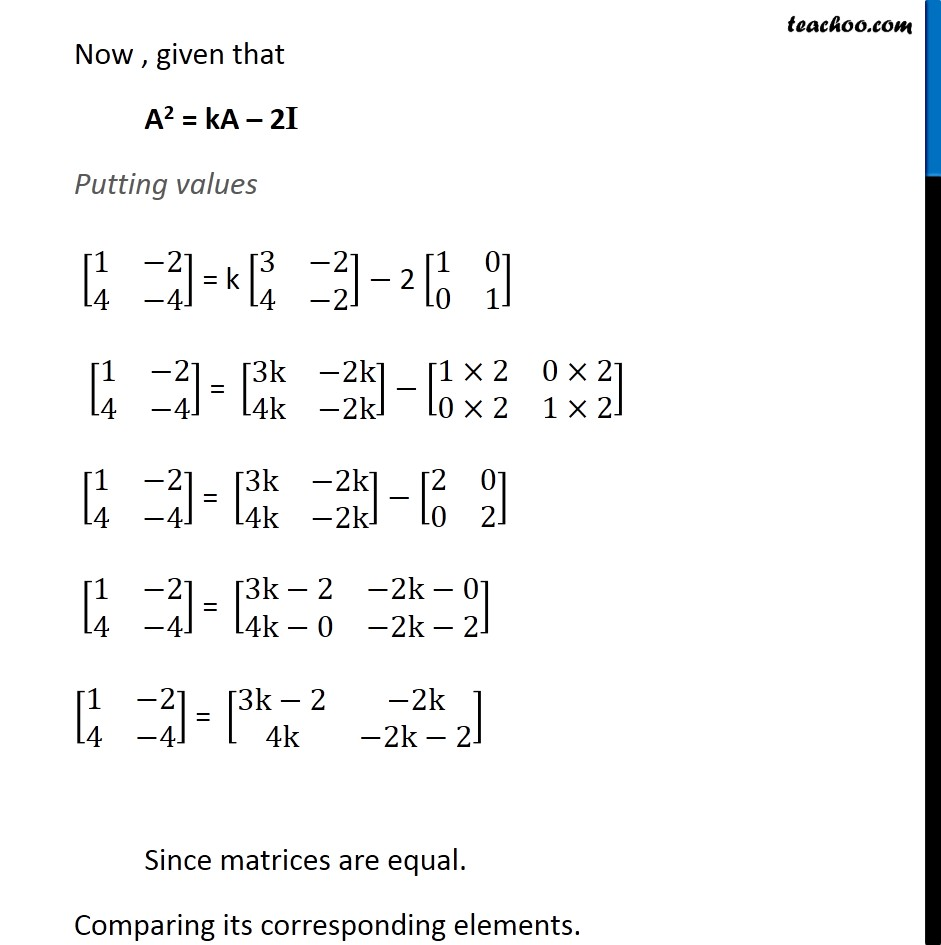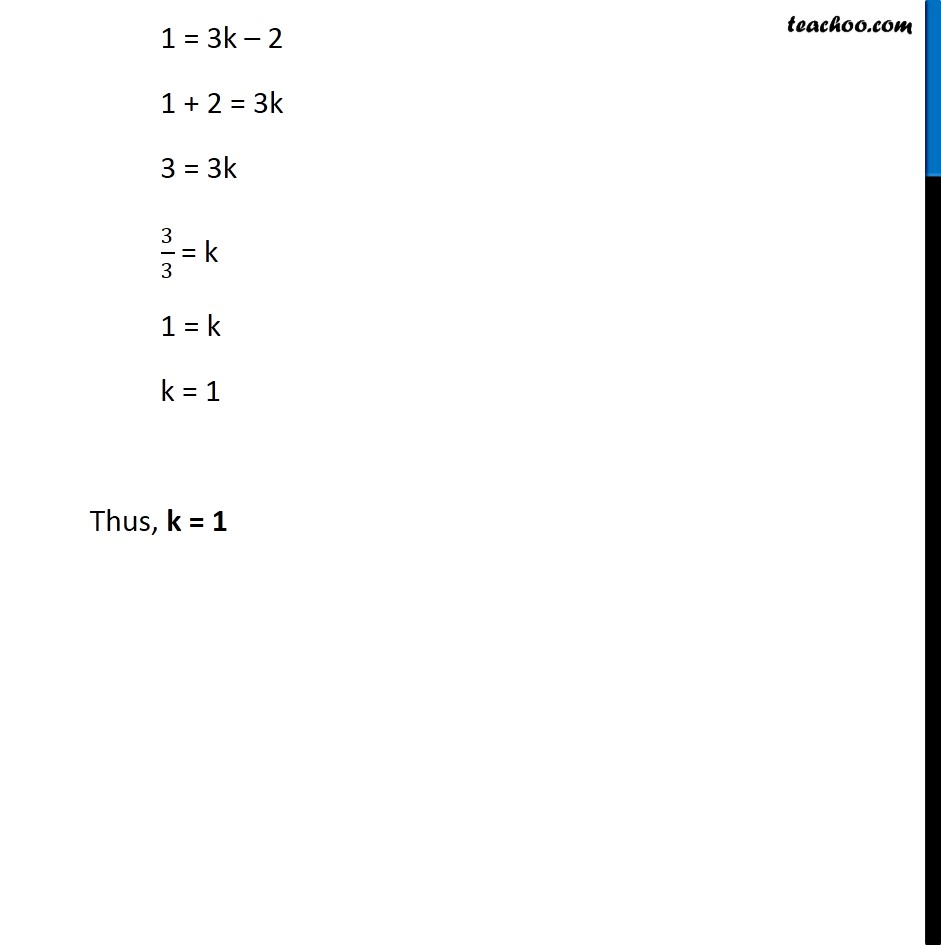1. Class 12
2. Important Questions for exams Class 12
3. Chapter 3 Class 12 Matrices

Transcript

Ex 3.2, 17 If A = [■8(3&−2@4&−2)] and I= [■8(1&0@0&1)] , find k so that A2 = kA – 2I Finding A2 A2 = A × A = [■8(3&−2@4&−2)][■8(3&−2@4&−2)] = [■8(3(3)+(−2)(4)&3(−2)+(−2)(−2)@4(3)+(−2)(4)&4(−2)+(−2)(−2))] = [■8(9−8&−6+4@12−8&−8+4)] = [■8(1&−2@4&−4)] ∴ A2 = [■8(1&−2@4&−4)] Now , given that A2 = kA – 2I Putting values [■8(1&−2@4&−4)] = k [■8(3&−2@4&−2)] − 2 [■8(1&0@0&1)] [■8(1&−2@4&−4)] = [■8(3k&−2k@4k&−2k)] − [■8(1×2&0×2@0×2&1×2)] [■8(1&−2@4&−4)] = [■8(3k&−2k@4k&−2k)] − [■8(2&0@0&2)] [■8(1&−2@4&−4)] = [■8(3k−2&−2k−0@4k−0&−2k−2)] [■8(1&−2@4&−4)] = [■8(3k−2&−2k@4k&−2k−2)] Since matrices are equal. Comparing its corresponding elements. 1 = 3k – 2 1 + 2 = 3k 3 = 3k 3/3 = k 1 = k k = 1 Thus, k = 1

Chapter 3 Class 12 Matrices

Class 12
Important Questions for exams Class 12